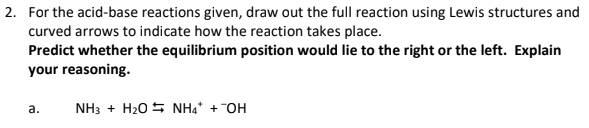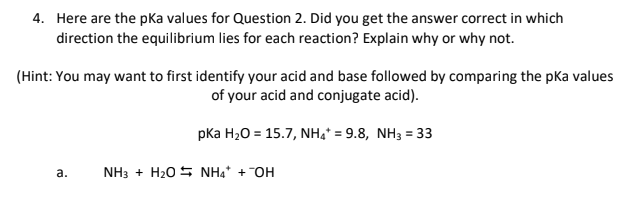# 2. For the acid-base reactions given, draw out the full reaction using Lewis structures and curved arrows to indicate how the reaction takes place. Predict whether the equilibrium position would lie to the right or the left. Explain your reasoning. a. NH3 + H2O ⇋ NH4^+ + --OH 4. Here are the pKa values for Question 2. Did you get the answer correct in which direction the equilibrium lies for each reaction? Explain why or why not. (Hint: You may want to first identify your acid and base followed by comparing the pKa values of your acid and conjugate acid). pKa H2O = 15.7, NH4^+ = 9.8, NH3 = 33 a. NH3 + H2O ⇋ NH4^+ + --OH